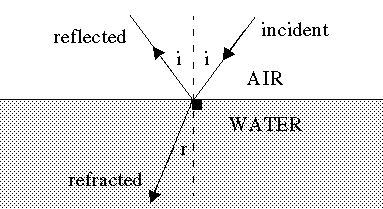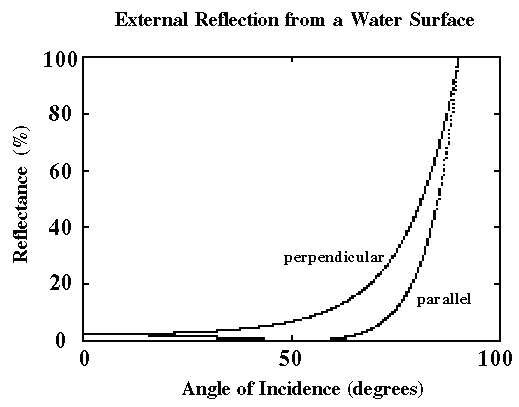External Reflection from a Water Surface

Light is a transverse electromagnetic wave, meaning that it consists of mutually perpendicular oscillatory electric and magnetic fields which are both perpendicular to the direction of propagation. In the most general case the electric field vector periodically changes direction and magnitude as it rotates around an ellipse, i.e. it is elliptically polarized. This can always be thought of as the superposition of two independent oscillations, with the same frequency, along mutually perpendicular lines with fixed orientations in space. Each of these oscillations is referred to as plane polarized, with the plane of polarization being the one containing both the line of oscillation and the direction of propagation.The application of Maxwell's equations of electrodynamics to this situation leads to rather simple expressions for the reflectances of waves with polarizations either perpendicular to or parallel to the plane of incidence. This is the plane containing the incident ray, reflected ray, refracted ray, and the normal to the surface at the point where the incident ray strikes it. In the figure shown below the reflectance for each direction of polarization has been plotted as a function of the angle of incidence.tan(i) = n(w)/n(a)